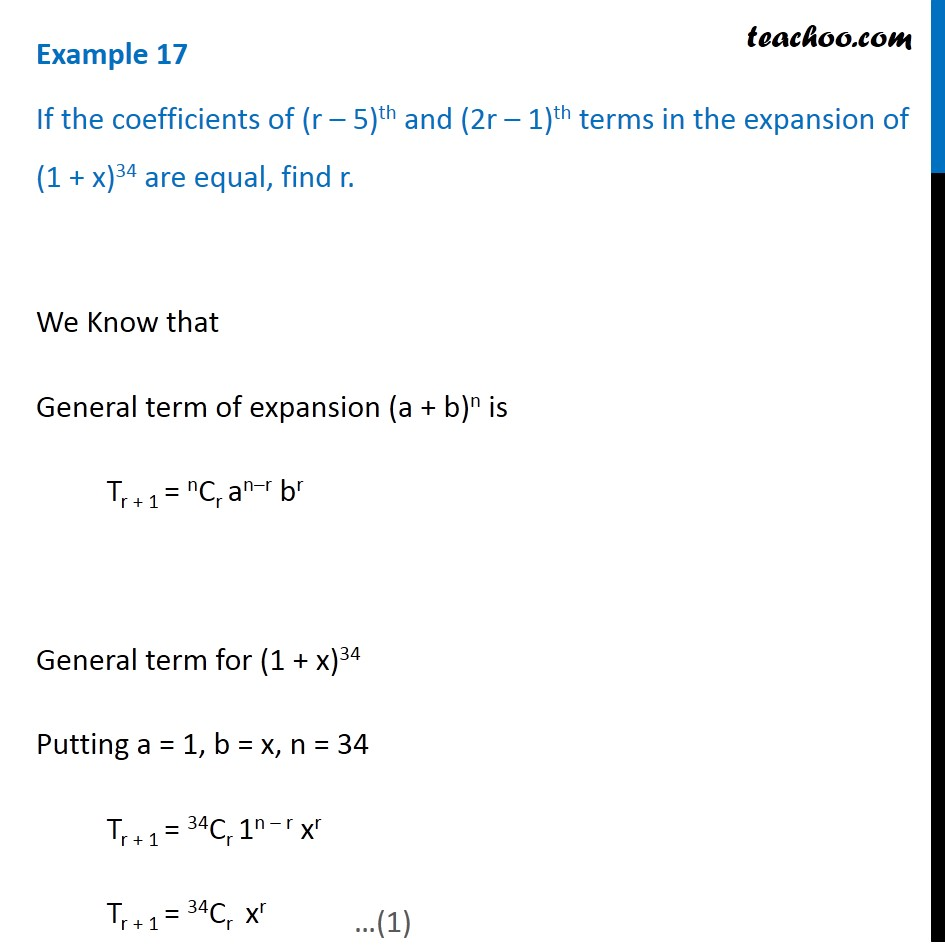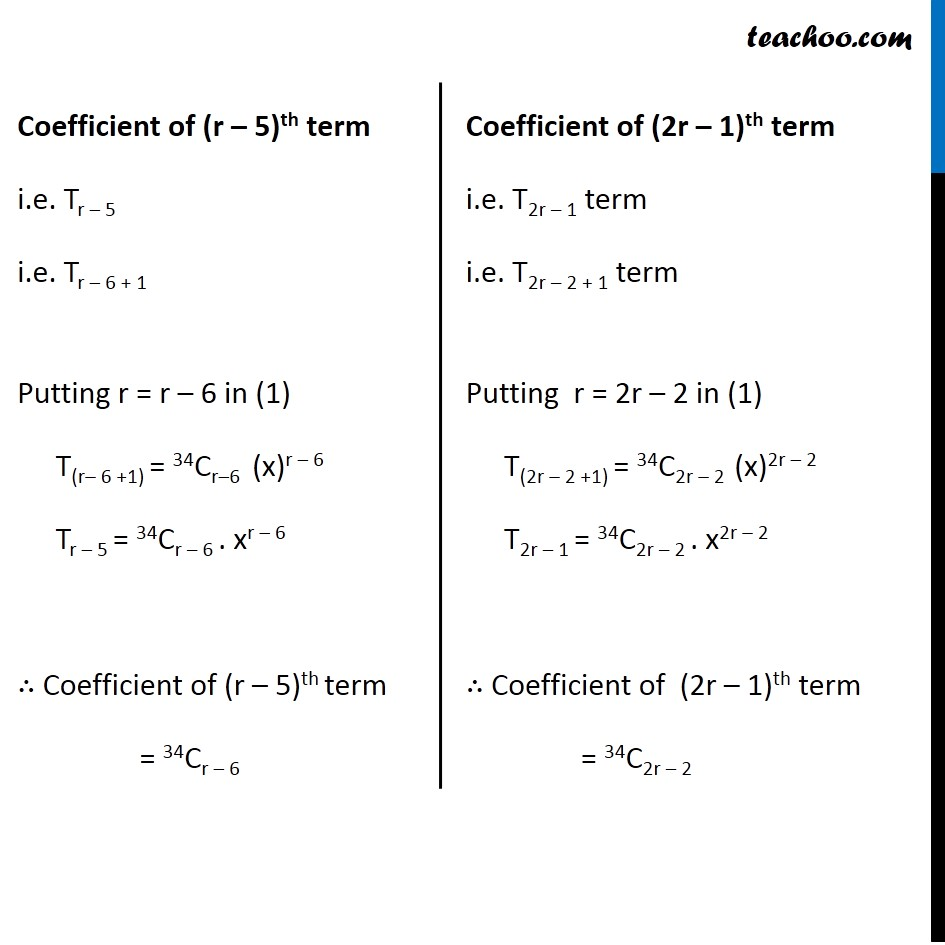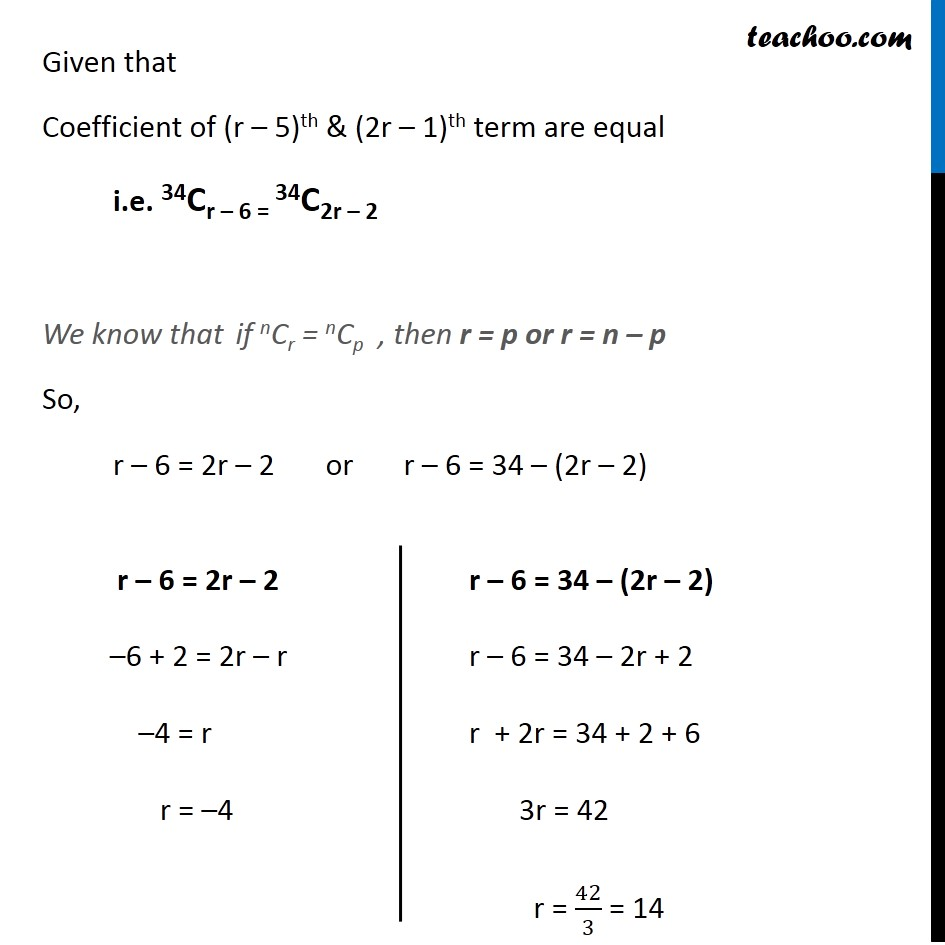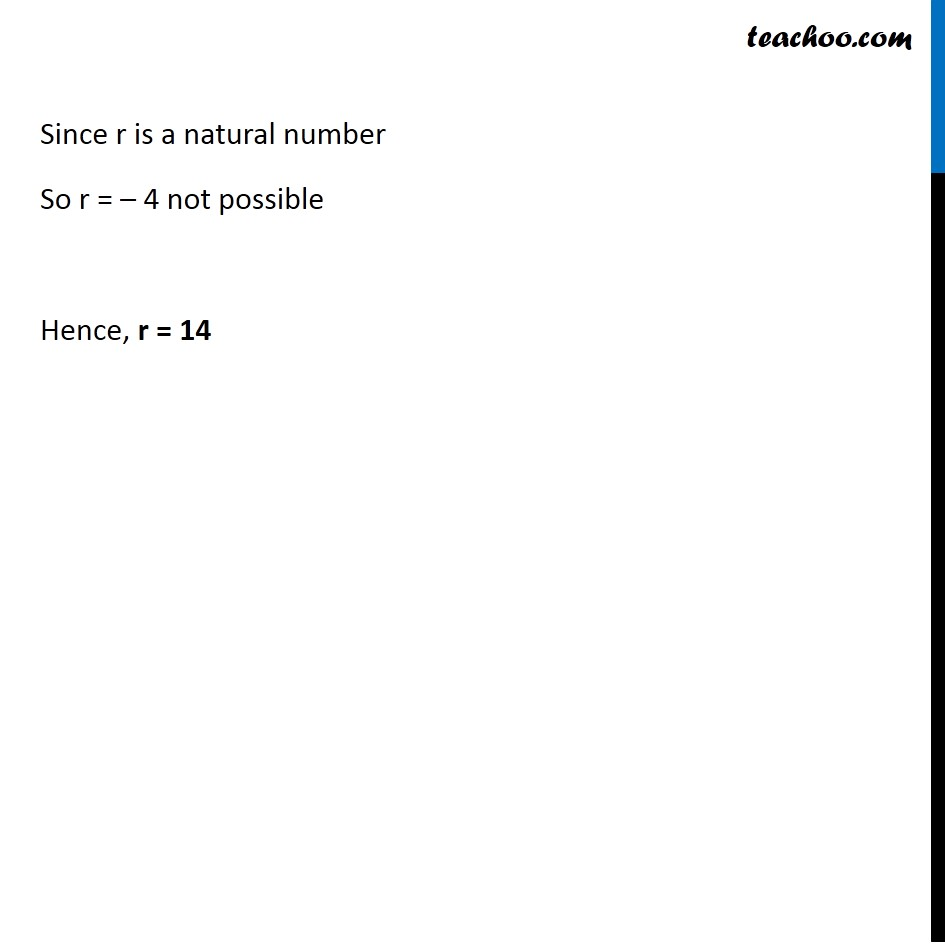Examples

Chapter 7 Class 11 Binomial Theorem
Serial order wiseLearn in your speed, with individual attention - Teachoo Maths 1-on-1 Class

### Transcript

Question 13 If the coefficients of (r – 5)th and (2r – 1)th terms in the expansion of (1 + x)34 are equal, find r. We Know that General term of expansion (a + b)n is Tr + 1 = nCr an–r br General term for (1 + x)34 Putting a = 1, b = x, n = 34 Tr + 1 = 34Cr 1n – r xr Tr + 1 = 34Cr xr Coefficient of (r – 5)th term i.e. Tr – 5 i.e. Tr – 6 + 1 Putting r = r – 6 in (1) T(r– 6 +1) = 34Cr–6 (x)r – 6 Tr – 5 = 34Cr – 6 . xr – 6 ∴ Coefficient of (r – 5)th term = 34Cr – 6 Coefficient of (2r – 1)th term i.e. T2r – 1 term i.e. T2r – 2 + 1 term Putting r = 2r – 2 in (1) T(2r – 2 +1) = 34C2r – 2 (x)2r – 2 T2r – 1 = 34C2r – 2 . x2r – 2 ∴ Coefficient of (2r – 1)th term = 34C2r – 2 Given that Coefficient of (r – 5)th & (2r – 1)th term are equal i.e. 34Cr – 6 = 34C2r – 2 We know that if nCr = nCp , then r = p or r = n – p So, r – 6 = 2r – 2 or r – 6 = 34 – (2r – 2) r – 6 = 2r – 2 –6 + 2 = 2r – r –4 = r r = –4 r – 6 = 34 – (2r – 2) r – 6 = 34 – 2r + 2 r + 2r = 34 + 2 + 6 3r = 42 r = 42/3 = 14 Since r is a natural number So r = – 4 not possible Hence, r = 14